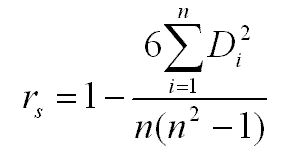The Spearman rank correlation coefficient rs is calculated as below:n is the number of pairs of measurements

Di is the difference of the ith pair of rankings